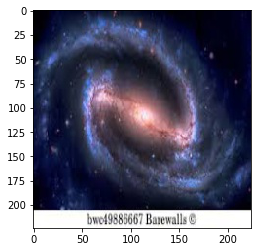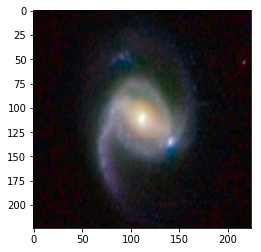# Trainsfer Learning with Azure Custom Vision¶

This notebook illustrates how to invoke the model created with the Azure Custom Vision portal https://customvision.ai. In the case here we have created the model as described in the accompaning blog post on https://esciencegroup.com. It consists of four classes of galaxy images with 19 samples in each class. We have a test set of 40 images with 10 from each class. We compute here the confusion matrix for the test set as well as the training set.

The first thing you need is two keys: Your prediction key and a project_id. You can get these going to the customvision portal for your project. there is a setting button on the upper right (small gear-shaped icon).

Of course you need the azure sdk installed with pip

In :
from azure.cognitiveservices.vision.customvision.prediction import prediction_endpoint
from azure.cognitiveservices.vision.customvision.training import training_api


Replace the keys below with your own. we are not going to train the network here, but we need the training key to access the list of iteration.

In :
# Replace with a valid key
training_key = 'aaab25your training key here 8a8b0'
prediction_key = "09199your prediction key here b9ae"

trainer = training_api.TrainingApi(training_key)
project_id = 'fcbccf40-1bce-4bc4-b4ea-025d63f1014d'

# Create a new project
print("Creating project...")
project = trainer.get_project(project_id)

Creating project...

In :
iterations = trainer.get_iterations(project.id)


During the training with this data we made 3 iterations. the first was with the initial data. The system recognized that one of the eliptical galaxies was a dupicate, so the second iteration included an additional eliptical galaxy. The system will not allow a new iteration until you have modified the data, so the third iteration repaced a randon spiral galaxy with another. We have selected the third iteration in the list

In :
iteration = iterations

In :
iteration.id

Out:
'8415c818-2349-4116-ba3b-a385efa827d8'

To test the predictor let's take a sample from the test set and display it and run the predictor on it

In :
import random
import matplotlib.pyplot as plt
import matplotlib.image as mpimg
%matplotlib inline
import json

In :
image_path = "https://s3-us-west-2.amazonaws.com/learn-galaxies/"
imagep = image_path+'bigtest/t5.jpg'

In :
import matplotlib.image as mpimg
from PIL import Image
import requests
from io import BytesIO
response = requests.get(imagep)
img = Image.open(BytesIO(response.content))
imgplot = plt.imshow(img)In :
predictor = prediction_endpoint.PredictionEndpoint(prediction_key)

In :
results = predictor.predict_image_url(project.id, iteration.id, url=imagep)

In :
for prediction in results.predictions:
print("\t" + prediction.tag + ": {0:.2f}%".format(prediction.probability * 100))

	barredspiral: 100.00%
spiral: 0.02%
irregular: 0.00%
elliptical: 0.00%

In :
def findbest(results):
best = 0
key = ''
for pred in results.predictions:
x = round(100*pred.probability, 2)
if x > best:
best = x
key = pred.tag
if key =='spiral galaxies':
key = 'spiral'
return key

In :
keys = ['spiral', 'barredspiral', 'elliptical', 'irregular']


Now let's compute the confusion matrix for the test set.

In :
mydict = {'spiral': {'spiral':0.0, 'barredspiral':0.0, 'elliptical':0.0, 'irregular':0.0},
'barredspiral': {'spiral':0.0, 'barredspiral':0.0, 'elliptical':0.0, 'irregular':0.0},
'elliptical': {'spiral':0.0, 'barredspiral':0.0, 'elliptical':0.0, 'irregular':0.0},
'irregular': {'spiral':0.0, 'barredspiral':0.0, 'elliptical':0.0, 'irregular':0.0}}

In :
base_image_url = "https://s3-us-west-2.amazonaws.com/learn-galaxies/"


In the test set, the first 10 items are barred spiral, the second 10 are eliptical, then 10 irregular followed by 10 spiral.

In :
print("doing barred spiral")
for i in range(0,10):
image_url = base_image_url + "bigtest/t{}.jpg".format(i)
try:
results = predictor.predict_image_url(project.id, iteration.id, url=image_url)
x = findbest(results)
mydict['barredspiral'][x]+= 1.
except:
print("-------------------")
print("doing elliptical")
for i in range(10,20):
image_url = base_image_url + "bigtest/t{}.jpg".format(i)
try:
results = predictor.predict_image_url(project.id, iteration.id, url=image_url)
x = findbest(results)
mydict['elliptical'][x]+= 1.
except:
print("-------------------")
print("doing irregular")
for i in range(20,30):
image_url = base_image_url + "bigtest/t{}.jpg".format(i)
try:
results = predictor.predict_image_url(project.id, iteration.id, url=image_url)
x = findbest(results)
mydict['irregular'][x]+= 1.
except:
print("-------------------")
print("doing spiral")
for i in range(30,40):
image_url = base_image_url + "bigtest/t{}.jpg".format(i)
try:
results = predictor.predict_image_url(project.id, iteration.id, url=image_url)
x = findbest(results)
mydict['spiral'][x]+= 1.
except:

doing barred spiral
-------------------
doing elliptical
-------------------
doing irregular
-------------------
doing spiral

In :
import pandas as pd

In :
df = pd.DataFrame.from_dict(mydict)

In :
df.transpose()

Out:
barredspiral elliptical irregular spiral
barredspiral 7.0 0.0 0.0 3.0
elliptical 2.0 8.0 0.0 0.0
irregular 1.0 2.0 6.0 1.0
spiral 1.0 0.0 0.0 9.0

Now compute the confusion matrix for the test set. do 19 tests for each type.

In :
train_mydict = {'spiral': {'spiral':0.0, 'barredspiral':0.0, 'elliptical':0.0, 'irregular':0.0},
'barredspiral': {'spiral':0.0, 'barredspiral':0.0, 'elliptical':0.0, 'irregular':0.0},
'elliptical': {'spiral':0.0, 'barredspiral':0.0, 'elliptical':0.0, 'irregular':0.0},
'irregular': {'spiral':0.0, 'barredspiral':0.0, 'elliptical':0.0, 'irregular':0.0}}

In :
print("doing barred spiral")
for i in range(1,20):
image_url = base_image_url + "bigbarred/bs{}.jpg".format(i)
try:
results = predictor.predict_image_url(project.id, iteration.id, url=image_url)
x = findbest(results)
train_mydict['barredspiral'][x]+= 1.
except:
print("-------------------")
print("doing elliptical")
for i in range(1,20):
image_url = base_image_url + "bigelliptical/e{}.jpg".format(i)
try:
results = predictor.predict_image_url(project.id, iteration.id, url=image_url)
x = findbest(results)
train_mydict['elliptical'][x]+= 1.
except:
print("-------------------")
print("doing irregular")
for i in range(1,20):
image_url = base_image_url + "bigirregular/i{}.jpg".format(i)
try:
results = predictor.predict_image_url(project.id, iteration.id, url=image_url)
x = findbest(results)
train_mydict['irregular'][x]+= 1.
except:
print("-------------------")
print("doing spiral")
for i in range(1,20):
image_url = base_image_url + "bigspiral/s{}.jpg".format(i)
try:
results = predictor.predict_image_url(project.id, iteration.id, url=image_url)
x = findbest(results)
train_mydict['spiral'][x]+= 1.
except:

doing barred spiral
-------------------
doing elliptical
-------------------
doing irregular
-------------------
doing spiral

In :
train_df = pd.DataFrame.from_dict(train_mydict)

In :
train_df.transpose()

Out:
barredspiral elliptical irregular spiral
barredspiral 18.0 0.0 0.0 1.0
elliptical 0.0 19.0 0.0 0.0
irregular 0.0 0.0 19.0 0.0
spiral 0.0 0.0 0.0 19.0

only got 1 wrong. not too bad. let's see which galaxy caused problems.

In :
for i in range(1,20):
image_url = base_image_url + "bigbarred/bs{}.jpg".format(i)
results = predictor.predict_image_url(project.id, iteration.id, url=image_url)
x = findbest(results)
if x != 'barredspiral':

bad one is 3